# How to Create a GUI Button in MATLAB App?

In MATLAB App environment, we can develop a GUI (Graphical User Interface) app without having proper knowledge of coding. Therefore, MATLAB app building allows us to create professional applications without writing code, and just by drag−drop.

In this article, we will discuss how to create a GUI button in a MATLAB application. The step−by−step procedure to create a GUI button in a MATLAB app is explained below.

## Steps to Create GUI Button in MATLAB App

We can follow the following steps to create a GUI button in a MATLAB application:

Step(1)– Open the MATLAB command window.

Step(2)– Launch the MATLAB App Designer.

There are the following two methods to open the app designer in MATLAB:

• Type ‘appdesigner’ command in the MATLAB command window and press enter.

• Click on the Design App option under the APPS tab.

Step (3)– In the App Designer window, select ‘New’ to create a new application. It will give a blank canvas to design a GUI app.

Step (4)– Drag and drop a “Button” from the Toolbox displayed on the left side of the App Designer window.

Step (5)– Customize the button as per your needs. For this, use the component browser on the right side of the App designer window to change the properties of the button.

Step (6)– Make the button functional by creating a callback function for the button. For this, right click on the button. Then, click on the ‘Callbacks’ option in the context menu. Then, click on “Add ButtonPushedFcn callback”. This will open a new command window in the MATLAB editor to write the callback function for the button.

Step (7)– Write a MATLAB code inside this function that you want to execute when the button is clicked.

Step (8)– Finally, save the app by clicking on the “Save” option in the App Designer.

Step (9)– Click on the “Run” option in the App Designer to execute the app. This app window will contain the button.

This is how, we can use the MATLAB app designer to create a GUI button in our MATLAB app.

Now, let us take an example to create a GUI button in MATLAB app to multiply two number when it is clicked.

## Create a GUI Button to Multiply Two Number in MATLAB App

The step−by−step process to create a GUI button in a MATLAB app to multiply two numbers when it is clicked is explained below:

Step (1)− Open MATLAB and launch the MATLAB App Designer in the APPS tab.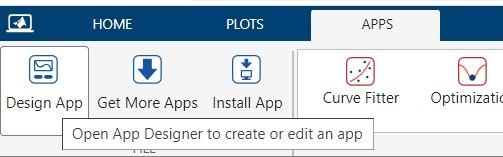Step (2)− Create a new bank app.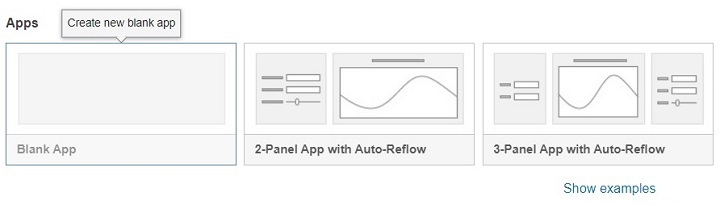Step (3)− Drag and drop the required components from the component library. In this example, we require the following components:

• Three Numeric Edit Fields: Two for inputting two numbers and one for getting product.

• A Button: To perform multiplication.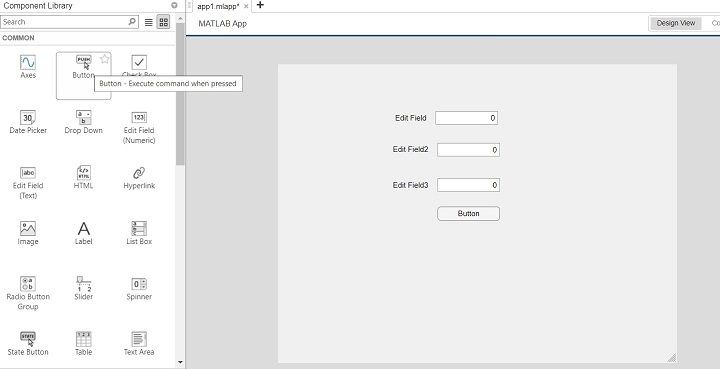Step (4)− Customize the components, such as add desired labels to fields and the button.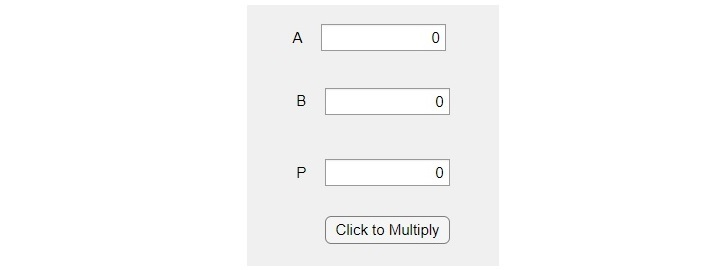Step (5)− Add the callback function to the button. For this, right click on the button, then click on the “callbacks”, and then click on “Add ButtonPushedFcn callback”. A new tab will open in the MATLAB editor to write codes.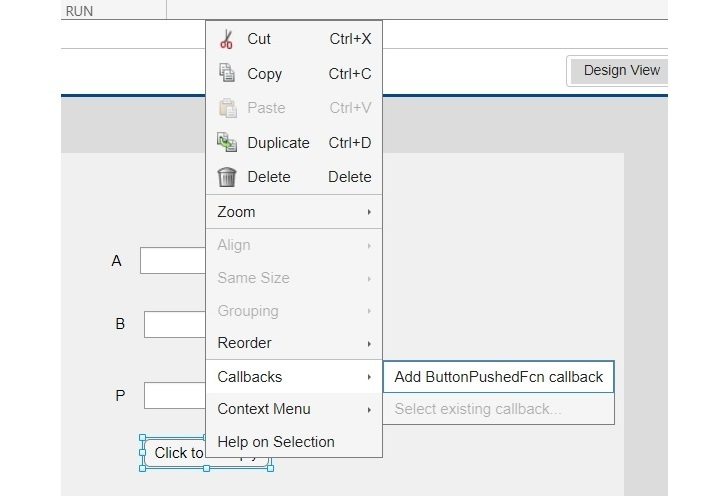Step (6)− Write the MATLAB code specifying the function of the button.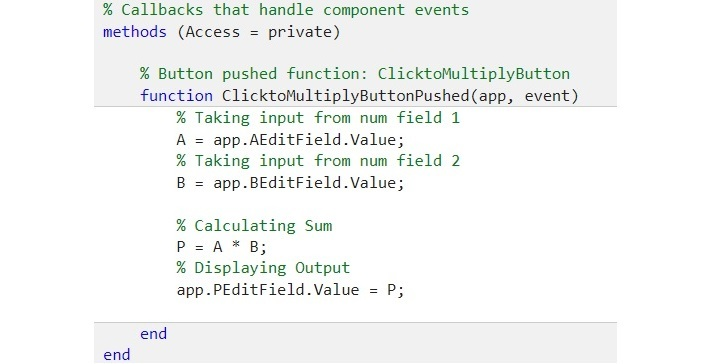For this example, we will write the following code:

% Callbacks that handle component events
methods (Access = private)

% Button pushed function: ClicktoMultiplyButton
function ClicktoMultiplyButtonPushed(app, event)
% Taking input from num field 1
A = app.AEditField.Value;
% Taking input from num field 2
B = app.BEditField.Value;

% Calculating Sum
P = A * B;
% Displaying Output
app.PEditField.Value = P;

end
end


Step (7)− Click on the “Save” button in the App designer to save the app.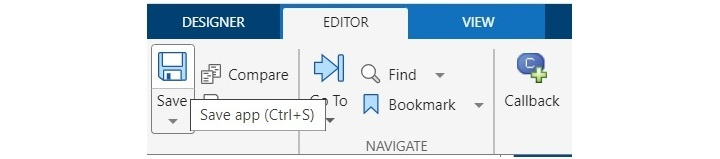Step (8)− Click on the “Run” button to execute the app.Step (9)− Input the parameters and click on the “Click to Multiply” button to execute the button’s function.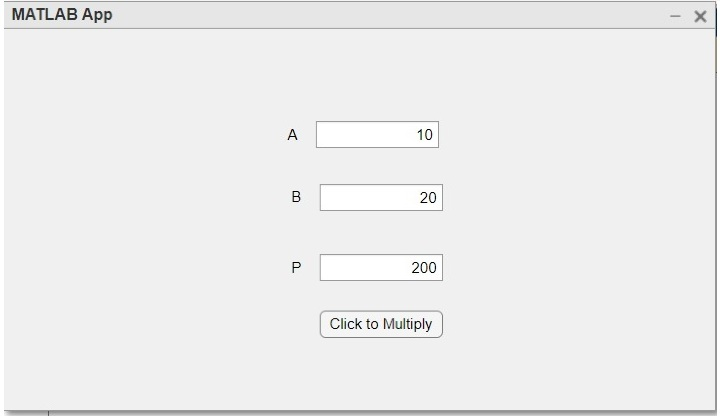This way, we can easily create a GUI button in MATLAB App using the MATLAB App Designer.

## MATLAB Code of Button

The following is the complete MATLAB code for the above GUI button app.

### Example

classdef product < matlab.apps.AppBase

% Properties that correspond to app components
properties (Access = public)
UIFigure               matlab.ui.Figure
ClicktoMultiplyButton  matlab.ui.control.Button
PEditField             matlab.ui.control.NumericEditField
PEditFieldLabel        matlab.ui.control.Label
BEditField             matlab.ui.control.NumericEditField
BEditFieldLabel        matlab.ui.control.Label
AEditField             matlab.ui.control.NumericEditField
AEditFieldLabel        matlab.ui.control.Label
end

% Callbacks that handle component events
methods (Access = private)

% Button pushed function: ClicktoMultiplyButton
function ClicktoMultiplyButtonPushed(app, event)
% Taking input from num field 1
A = app.AEditField.Value;
% Taking input from num field 2
B = app.BEditField.Value;

% Calculating Sum
P = A * B;
% Displaying Output
app.PEditField.Value = P;

end
end

% Component initialization
methods (Access = private)

% Create UIFigure and components
function createComponents(app)

% Create UIFigure and hide until all components are created
app.UIFigure = uifigure('Visible', 'off');
app.UIFigure.Position = [100 100 640 480];
app.UIFigure.Name = 'MATLAB App';

% Create AEditFieldLabel
app.AEditFieldLabel = uilabel(app.UIFigure);
app.AEditFieldLabel.HorizontalAlignment = 'right';
app.AEditFieldLabel.Position = [214 384 25 22];
app.AEditFieldLabel.Text = 'A';

% Create AEditField
app.AEditField = uieditfield(app.UIFigure, 'numeric');
app.AEditField.Position = [254 384 100 22];

% Create BEditFieldLabel
app.BEditFieldLabel = uilabel(app.UIFigure);
app.BEditFieldLabel.HorizontalAlignment = 'right';
app.BEditFieldLabel.Position = [217 333 25 22];
app.BEditFieldLabel.Text = 'B';

% Create BEditField
app.BEditField = uieditfield(app.UIFigure, 'numeric');
app.BEditField.Position = [257 333 100 22];

% Create PEditFieldLabel
app.PEditFieldLabel = uilabel(app.UIFigure);
app.PEditFieldLabel.HorizontalAlignment = 'right';
app.PEditFieldLabel.Position = [217 276 25 22];
app.PEditFieldLabel.Text = 'P';

% Create PEditField
app.PEditField = uieditfield(app.UIFigure, 'numeric');
app.PEditField.Position = [257 276 100 22];

% Create ClicktoMultiplyButton
app.ClicktoMultiplyButton = uibutton(app.UIFigure, 'push');
app.ClicktoMultiplyButton.ButtonPushedFcn = createCallbackFcn(app, @ClicktoMultiplyButtonPushed, true);
app.ClicktoMultiplyButton.Position = [257 230 100 22];
app.ClicktoMultiplyButton.Text = 'Click to Multiply';

% Show the figure after all components are created
app.UIFigure.Visible = 'on';
end
end

% App creation and deletion
methods (Access = public)

% Construct app
function app = product

% Create UIFigure and components
createComponents(app)

% Register the app with App Designer
registerApp(app, app.UIFigure)

if nargout == 0
clear app
end
end

% Code that executes before app deletion
function delete(app)

% Delete UIFigure when app is deleted
delete(app.UIFigure)
end
end
end


### Output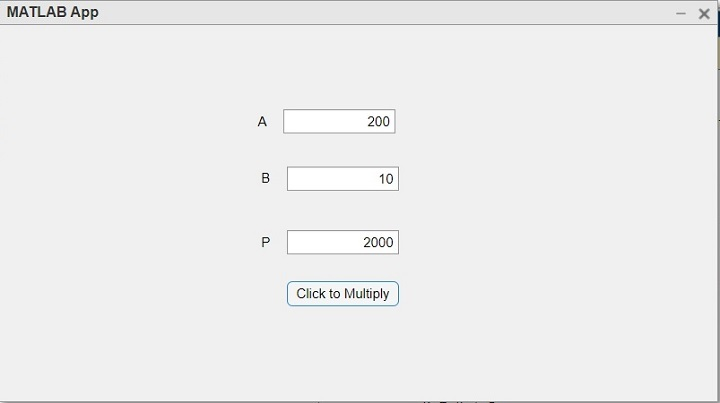## Conclusion

Hence, this is all about creating a GUI button in MATLAB app. In this article, we have explained the steps to create a GUI button in a MATLAB app. Also, we have explained the same with the help of an example, where we have created a button to multiply two numbers in a MATLAB app.

Updated on: 08-Aug-2023

100 Views